• 机器学习聚类分析
2020-10-16 16:06:37

## 聚类

监督学习：线性回归 逻辑回归 KNN（这里引入KNN是为了与Kmeans进行区分）

非监督学习：聚类 (Kmeans Mean-shit)

## 无监督学习(unsupervised learning)

无监督学习:机器学习的一种方法，没有给定事先标记过的训练实例，自动对输入的数据进行分类或分群

优点：

• 算法不受监督信息（偏见）的约束，可能考虑到新的信息
• 不需要标签，极大程度扩大数据样本

主要应用： 聚类分析、关联规则、维度缩减

聚类分析(clustering):聚类分析又称为群分析，根据对象某些属性的相似度，将其自动划分为不同的类别。

## KMeans聚类

KMeans Analysis: 以空间中k个点为中心进行聚类，对最靠近他们的对象归类，是聚类算法中最为基础但也最为重要的算法。

过程：

• 根据数据与中心点距离划分类别
• 基于类别数据更新中心点
• 重复过程直到收敛

公式算法流程：

• 选取聚类个数k
• 确定聚类中心
• 根据点到聚类中心聚类确定各个点所属类别
• 根据各个类别数据数据更新聚类中心
• 重复以上步骤直到收敛（中心点不再变化）

代码实现

# 模型训练
from sklearn.cluster import KMeans
KM = KMeans(n_cluster = 3, random_state = 0)
KM.fit(X)

#获取模型确定的中心点
centers = KM.cluster_centers_

#准确率计算
from sklearn.metrics import accuracy_score
accuracy = accuracy_score(y,y_predict)

#预测结果矫正
y_cal = []
for i in y_predict:
if i == 0:
y_cal.append(2)
elif i == 1:
y_cal.append(1)
else:
y_cal.append(0)
print(y_predict,y_cal)



特点：

• 优：原理简单，实现容易，收敛速度快
• 优：参数少，方便使用（需要指定类别数量
• 缺：必须设置簇的的数量
• 缺：随机选择初始聚类中心，结果可能缺乏一致性

## K近邻分类模型(KNN)

KNN:给定一个训练数据集，对新的输入实例，在训练数据集中找到与该实例最邻近的K个实例（也就是K个邻居），这k个实例的多数属于某个类，就把该输入实例分类到这个类中。是最简单的机器学习算法之一。

代码实现

#模型训练
from sklearn.neighbors import KNeighborsClassifier
KNN = KNeighborsClassifier(n_neighbors = 3)
KNN.fit(X,y)


## 均值漂移聚类（Meanshift）

Meanshift: 一种基于密度梯度上升的聚类算法（沿着密度上升方向寻找聚类中心点）

过程：

• 随机均匀的选取样本点
• 在中心点一定区域检索数据点
• 更新数据中心
• 重复流程到中心点稳定

公式：算法流程：

• 随机选择未分类点作为中心点
• 找出离中心点距离在带宽之内的点，记作集合S
• 计算从中心点到集合S中每个元素的偏移向量M
• 中心点以向量M移动
• 重复步骤2-4，直到收敛
• 重复1-5直到所有的点都被归类
• 分类：根据每个类，对每个点的访问频率，取访问频率最大的那个类，作为当前点集的所属类

代码实现

# 自动计算带宽（区域半径）
from sklearn.cluster import
MeanShift,estimate_bandwidth
#detect bandwidth
bandwidth = estimate_bandwidth(X,n_samples=500)

# 模型建立与训练
ms = MeanShift(bandwidth = bandwidth)
ms.fit(X)


特点：

• 自动发现类别数量，不需要人工选择
• 需要选择区域半径

## DBSCAN算法（基于密度的空间聚类算法）

过程

• 基于区域点密度筛选有效数据
• 基于有效数据向周围扩张，直到没有新点加入

特点

• 过滤噪声数据
• 不需要认为选择类别数量
• 数据密度不同时影响结果机器学习 python 深度学习 聚类 算法
更多相关内容
• 聚类是基于谱图理论基础上的一种聚类方法，与传统的聚类方法相比：具有在任意形状的样本空间上聚类并且收敛于全局最优解的优点。（但效率不高，实际工作中用的比较少） 谱聚类 通过对样本数据的拉普拉斯矩阵的特征...作者：韩信子@ShowMeAI
教程地址http://www.showmeai.tech/tutorials/34
本文地址http://www.showmeai.tech/article-detail/197
声明：版权所有，转载请联系平台与作者并注明出处

# 引言

聚类（Clustering）是最常见的无监督学习算法，它指的是按照某个特定标准（如距离）把一个数据集分割成不同的类或簇，使得同一个簇内的数据对象的相似性尽可能大，同时不在同一个簇中的数据对象的差异性也尽可能地大。也即聚类后同一类的数据尽可能聚集到一起，不同类数据尽量分离。

聚类算法在很多场景下都有应用，例如新闻自动分组，用户分群，图像分割等等。很多时候，无监督的聚类算法，得到的聚类结果还可以作为特征在后续监督学习中应用，提升整体效果。本篇内容ShowMeAI带大家一起来学习一下聚类算法。

（本篇聚类算法部分内容涉及到机器学习基础知识，没有先序知识储备的宝宝可以查看ShowMeAI的文章 图解机器学习 | 机器学习基础知识

# 1.聚类问题

## 1）聚类问题与核心概念

俗话说人以类聚物以群分，聚类算法做的事情，就是对无标签的数据，基于数据分布进行分群分组，使得相似的数据尽量落在同一个簇内。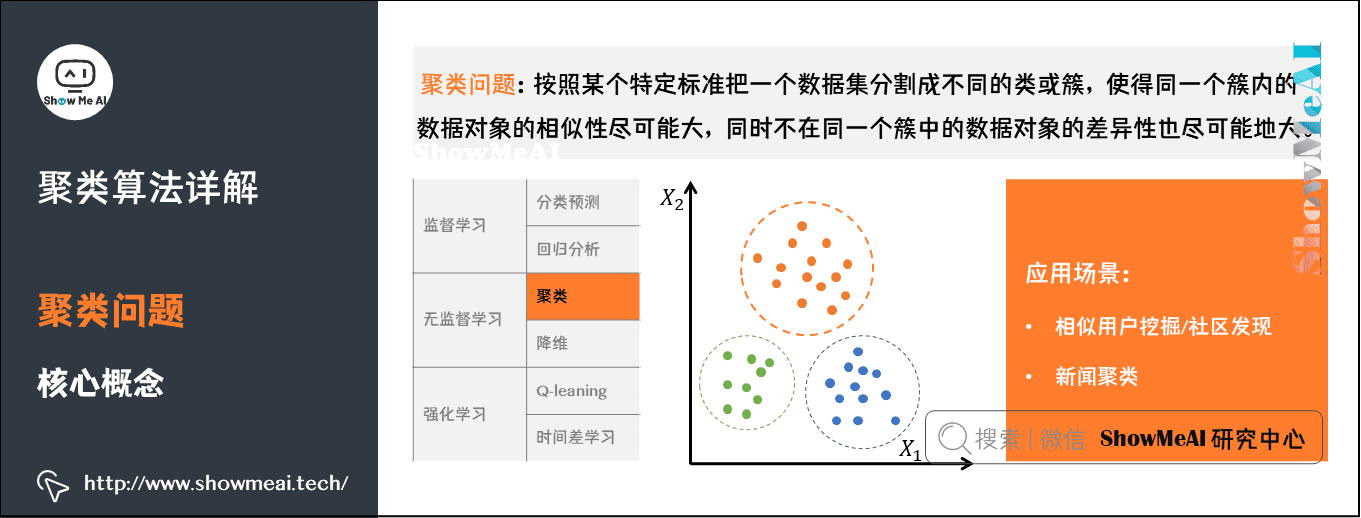我们先对比区分一下聚类和分类

• 聚类是一种无监督学习，而分类是一种有监督的学习。
• 聚类只需要人工指定相似度的标准和类别数就可以，而分类需要从训练集学习分类的方法。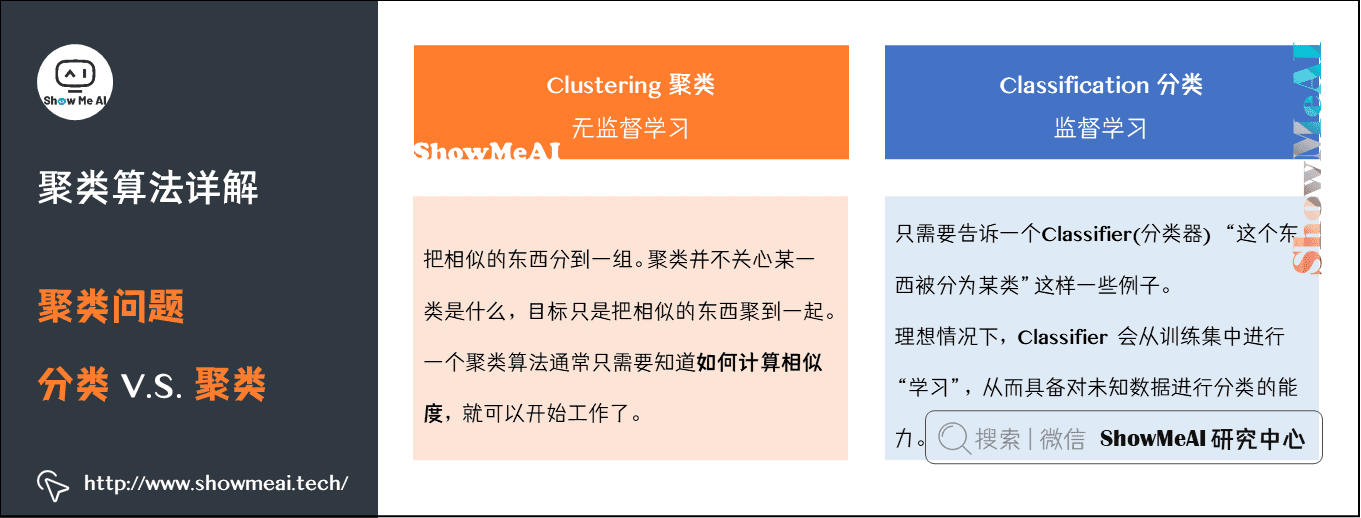## 2）聚类算法用途

聚类算法应用非常广泛。在面对未知的世界时，先分类，再逐个研究，是人类探索未知世界的一个基本方法。聚类算法可以应用于探索性数据挖掘、统计分析、生物信息学、数据压缩、计算机图像识别、医学影像分析等，在商业领域可以用来做市场研究、商品归类，在社会科学领域可以用来做犯罪区域分析等等。

下图中有一些样本点，我们根据物理距离的远近，把所有的点分成3类。你只需要告诉算法这些信息，算法就可以按照你的要求完成聚类：

• 分类数量为3；
• 分类标准是物理距离；
• 分好的类分别用红、绿、蓝表示。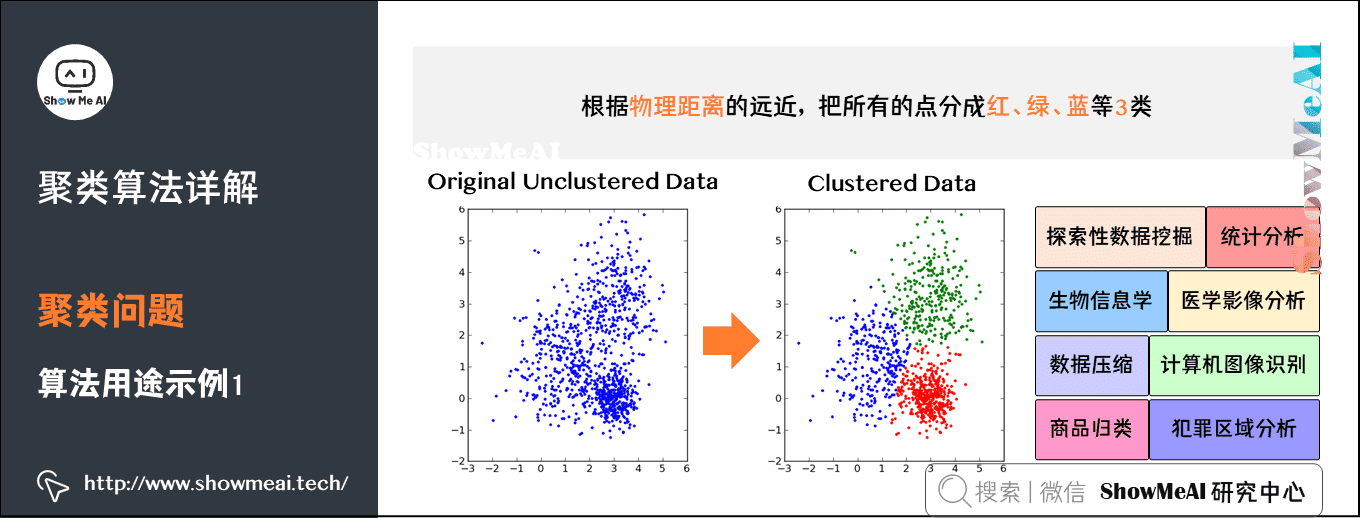实际上，除了物理距离，现实生活中任何你能想到、计算机可以通过运算和逻辑进行判断的规则，都可以作为分类标准

下面我们用图像压缩作为例子来解释一下。最左边是一张彩色照片，大小约1Mb，通过压缩可以把它变成几十到几百Kb，这就是压缩软件的实现过程。那么压缩软件的实现原理是什么呢？其中一种就是聚类算法。

从原始图片到压缩存储的过程如下图所示：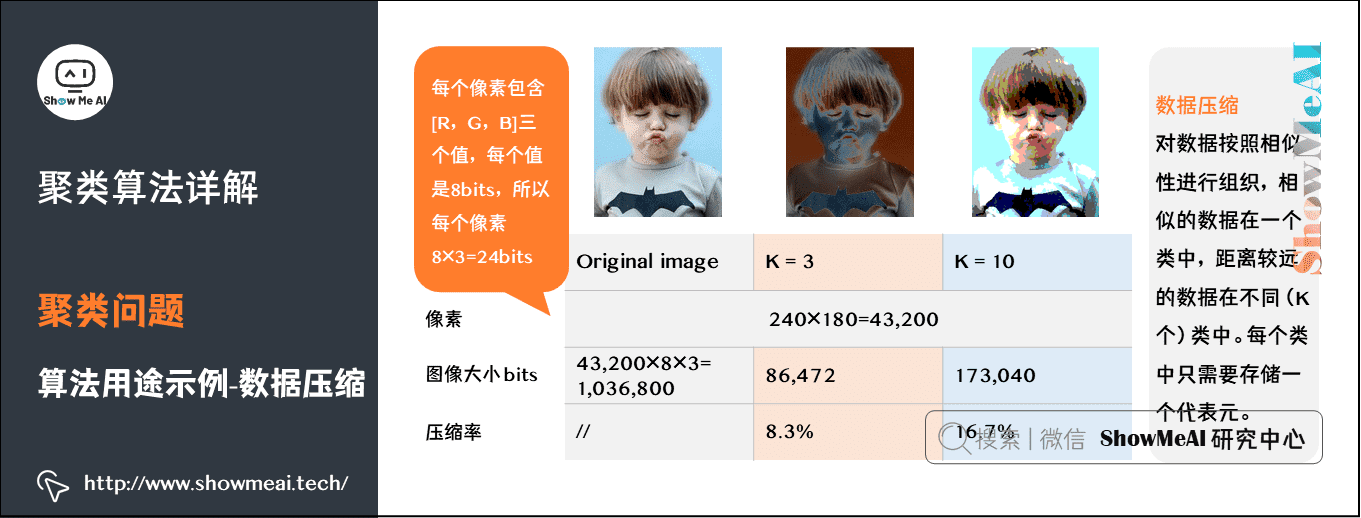聚类算法同样可以用于图像分割。图像中每一个像素点是一个3维向量，对应 [R, G, B] 像素值。给定聚类中类别个数K，算法用K个不同的颜色来表示原来的图像，每个像素点用K个颜色中一个表示。具体如下：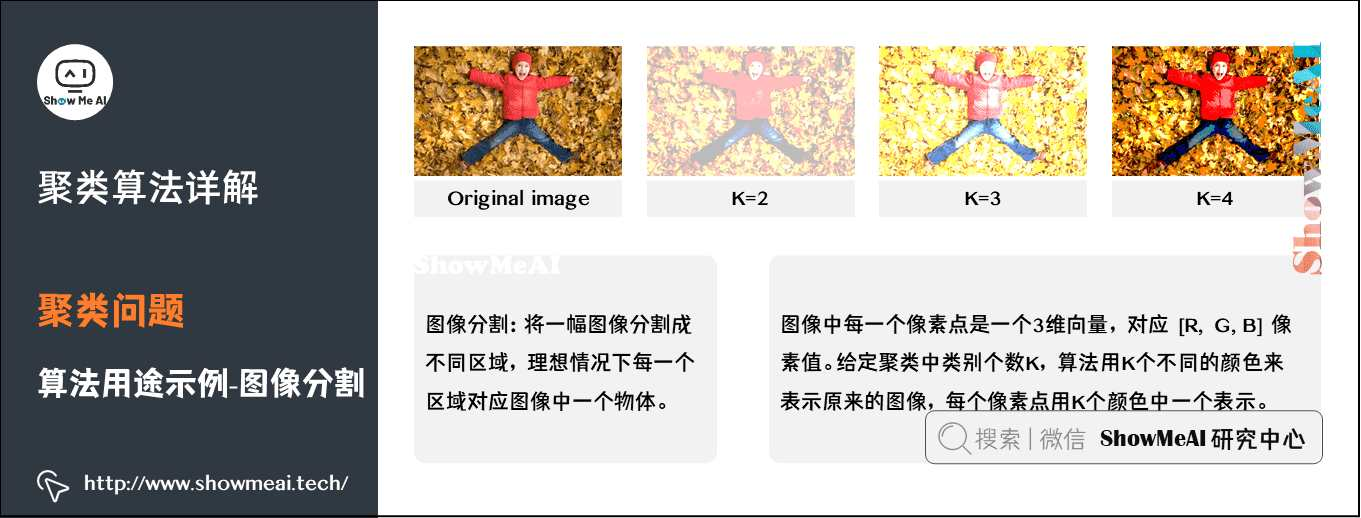对于文档、新闻、商品而言，很多时候我们会使用嵌套的归类方法，这是一种层次化聚类：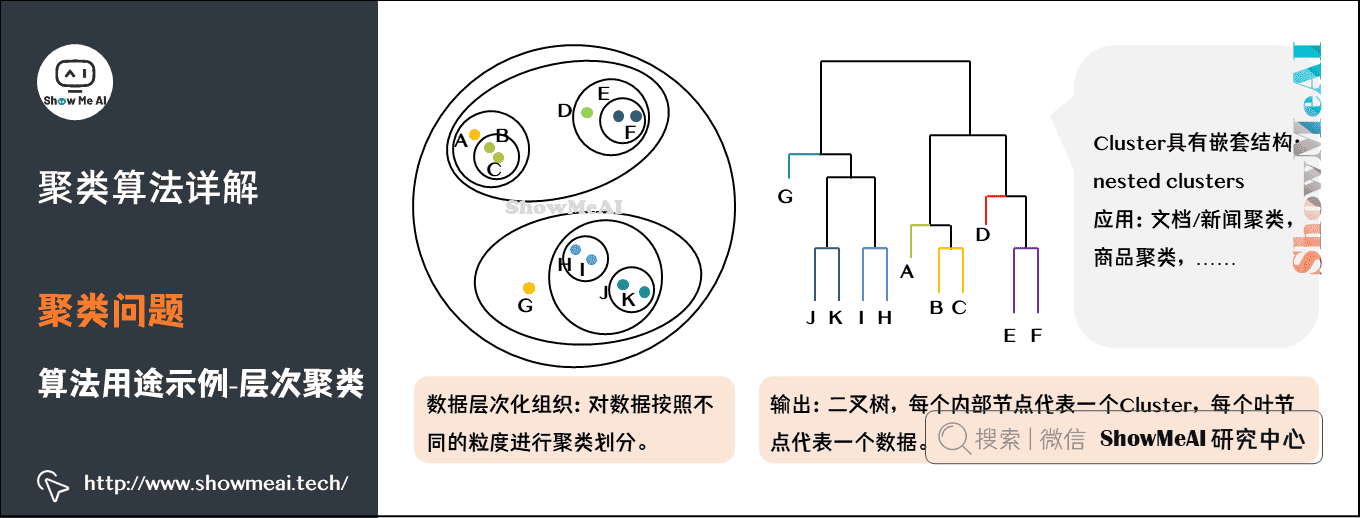## 3）主流聚类算法

我们先对聚类算法做个了解，主流的聚类算法可以分成两类：划分聚类（Partitioning Clustering）和层次聚类（Hierarchical Clustering）。他们的主要区别如图中所示：

划分聚类算法会给出一系列扁平结构的簇（分开的几个类），它们之间没有任何显式的结构来表明彼此的关联性

• 常见算法有 K-Means/K-Medoids、Gaussian Mixture Model （高斯混合模型）、Spectral Clustering（谱聚类）、Centroid-based Clustering等。

层次聚类会输出一个具有层次结构的簇集合，因此能够比划分聚类输出的无结构簇集合提供更丰富的信息。层次聚类可以认为是是嵌套的划分聚类。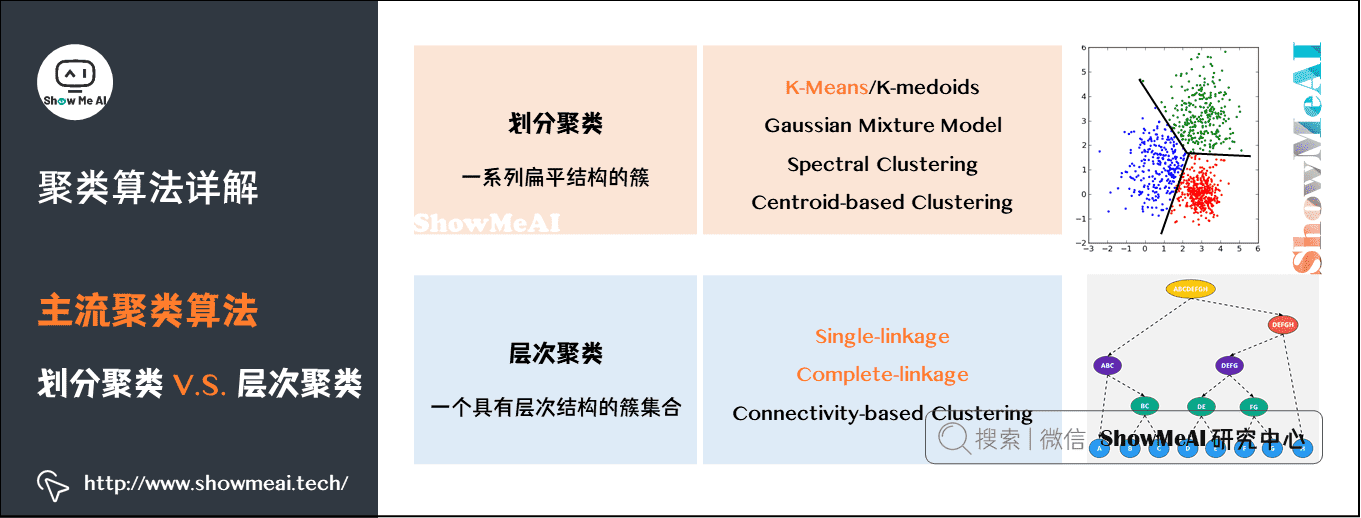# 2.K-Means聚类算法

K-Means算法是聚类算法中一个非常基础的算法，同时应用又非常广泛，下面ShowMeAI给大家展开讲解算法原理。

## 1）K-Means算法核心概念

我们提到了聚类算法要把n个数据点按照分布分成k类（很多算法的k是人为提前设定的）。我们希望通过聚类算法得到k个中心点，以及每个数据点属于哪个中心点的划分。

• 中心点可以通过迭代算法来找到，满足条件：所有的数据点到聚类中心的距离之和是最小的。

• 中心点确定后，每个数据点属于离它最近的中心点。

在进入「如何寻找中心点」这个核心问题之前，我们先解决几个小问题：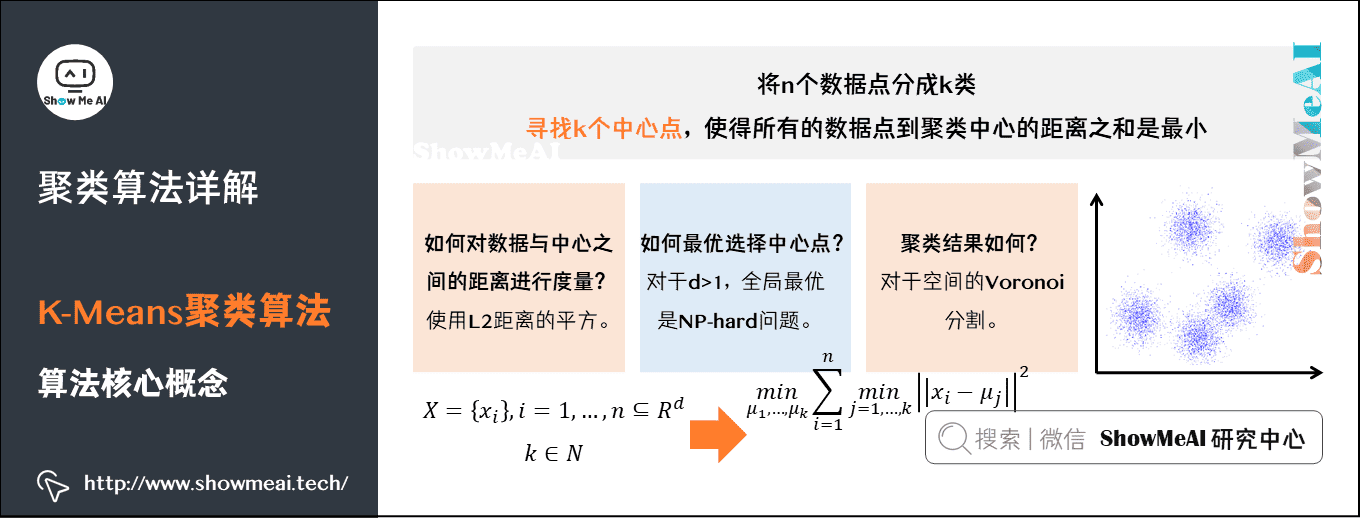Q1：数据点到中心点的距离如何计算

• 我们一般选择几何距离，就是L2距离的平方。

Q2：中心点是否唯一，或者说，是不是存在全局最优解

• 对于多个中心点的情况，全局最优是一个相当难的问题。理论上存在一个全局最优解，但是不一定能找到。既然全局最优解不好找，那我们退而求其次，看能不能找到局部最优解。

Q3：聚类结果如何表示

• 采用空间分割的方式：将空间分割成多个多边形，每个多边形对应一个cluster中心。

## 2）K-Means算法步骤

K-Means采用EM算法迭代确定中心点。流程分两步：

• ① 更新中心点：初始化的时候以随机取点作为起始点；迭代过程中，取同一类的所有数据点的重心（或质心）作为新中心点。

• ② 分配数据点：把所有的数据点分配到离它最近的中心点。

重复上面的两个步骤，一直到中心点不再改变为止。过程如图所示：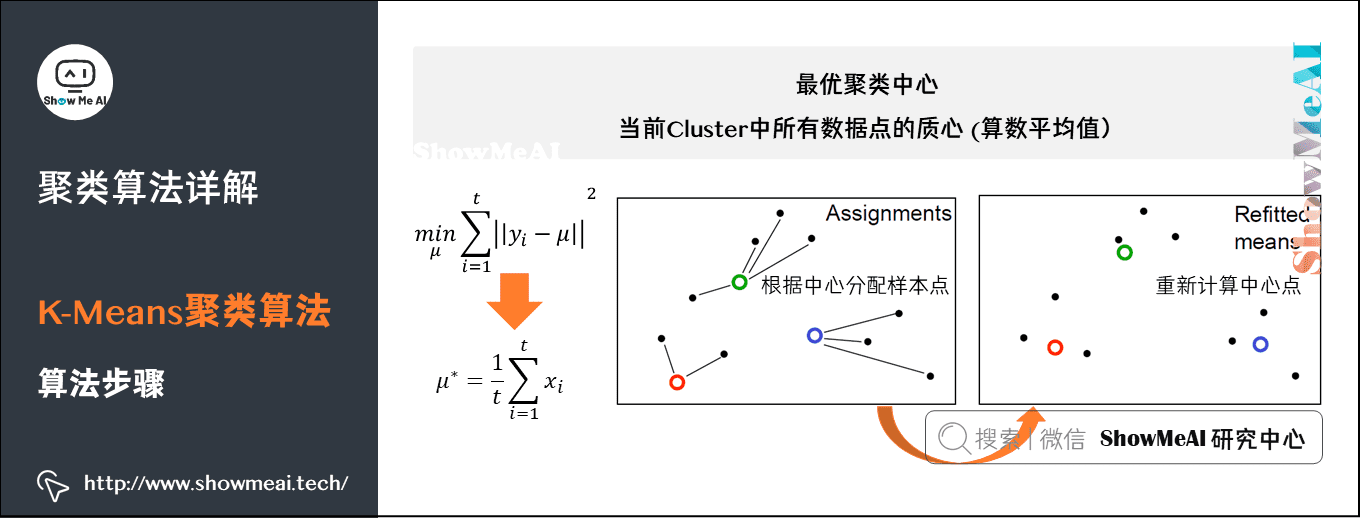• 左侧Assignments：一开始随机选取三个点，作为三个类的中心，基于其它点和这三个中心点的距离分配簇；每一类重新计算和分配中心。

• 右侧Refitted Means：根据新的中心点，重新分配所有的数据点（原来属于绿色中心点的1个点，此次迭代后变成属于红色中心点了）。

下图的示例展示了K-Means动态迭代收敛的过程：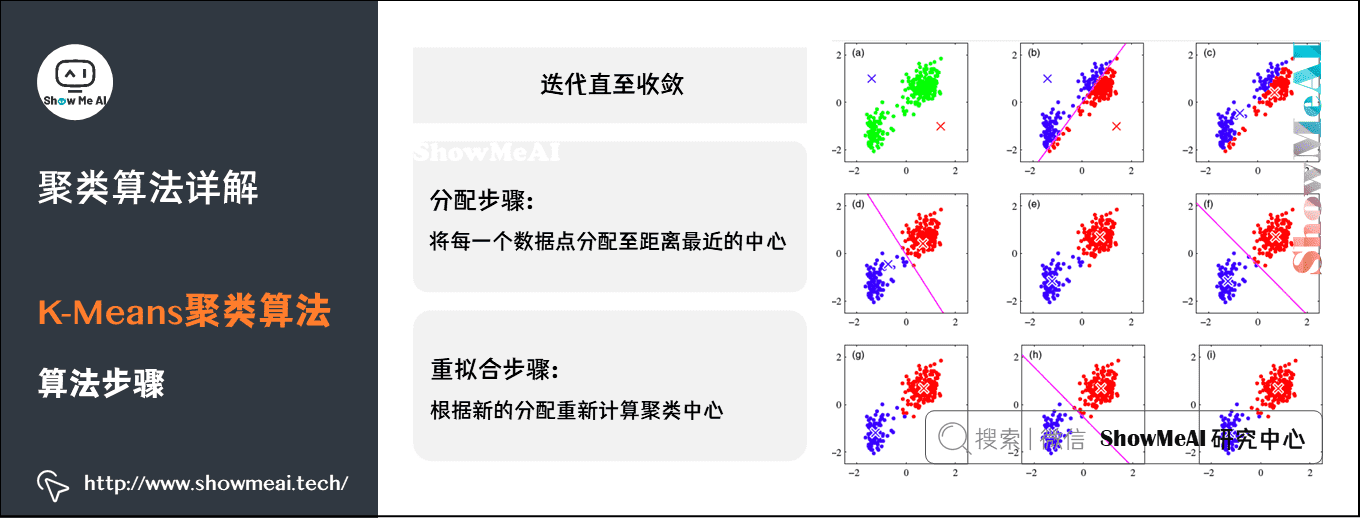• 图（a）上有一群散落的点，我们设定簇数K=2。

• 图（b）为随机找2个点作为中心初始化后，第一次分类的结果。

• 可以看到，红蓝分界线在这群点的中央穿过。这显然有问题，不过没关系，算法继续往下走。对红蓝两类分别计算它们的中心。
• 图（c）可以看到，一个落在左下方这一团里，另一个落在右上方那一团里。以新的中心点进行第二次分类。

• 图（d）的分界线就基本是已经可以把两团分开了。

• 图（f）、（g）显示后续重复计算你「中心点-分类数据点」的过程已经收敛，数据点分配基本不动了，聚类完成。

下方的动图能更清晰地展示这个过程：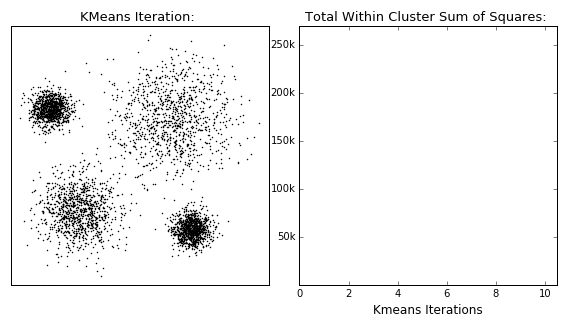# 3.K-Means缺点与改进

## 1）K-Means算法缺点

K-Means算法简单易用，它有什么缺点呢？我们将K-Means算法的一些缺点总结如下：

• 缺点1：中心点是所有同一类数据点的质心，所以聚类中心点可能不属于数据集的样本点。

• 缺点2：计算距离时我们用的是L2距离的平方。对离群点很敏感，噪声（Noisy Data）和离群点（Outlier）会把中心点拉偏，甚至改变分割线的位置。

## 2）K-Medoids算法

针对K-Means算法的缺点改进得到了K-Medoids算法：

### （1）限制聚类中心点必须来自数据点。

• 求中心点的计算方法，由原来的直接计算重心，变成计算完重心后，在重心附近找一个数据点作为新的中心点。

• K-Medoids重拟合步骤比直接求平均的K-Means要复杂一些。

### （2）为避免平方计算对离群点的敏感，把平方变成绝对值。

总结来说，K-Medoids算法的迭代过程与K-Means是一致的，不同点如下所示：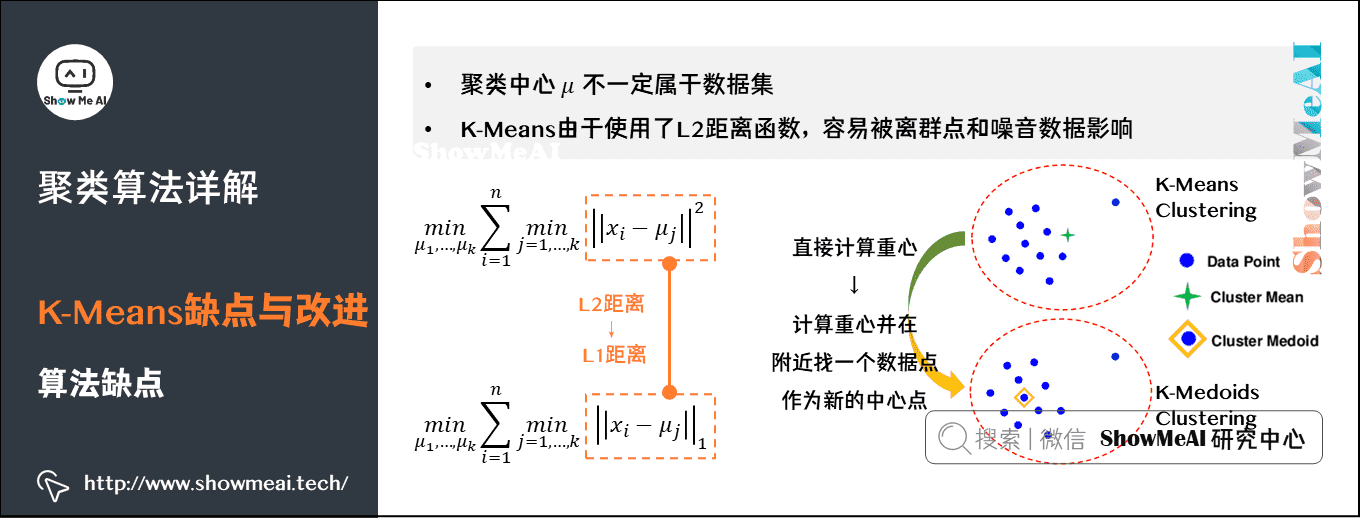• 起始点不是随机点，而是任意选择数据集中的点。

• 距离使用L1距离，而不是L2距离。

• 新的中心点，也不是同类所有点的重心，而是同一类别所有数据点中，离其它点最近的点。

• 复杂度方面，相比于K-Means的 O ( n ) O(n) ，K-Medoids更新中心点的复杂度 O ( n 2 ) O(n^2) 要更高一些。

下图是K-Means和K-Medoids两个算法的一个系统对比：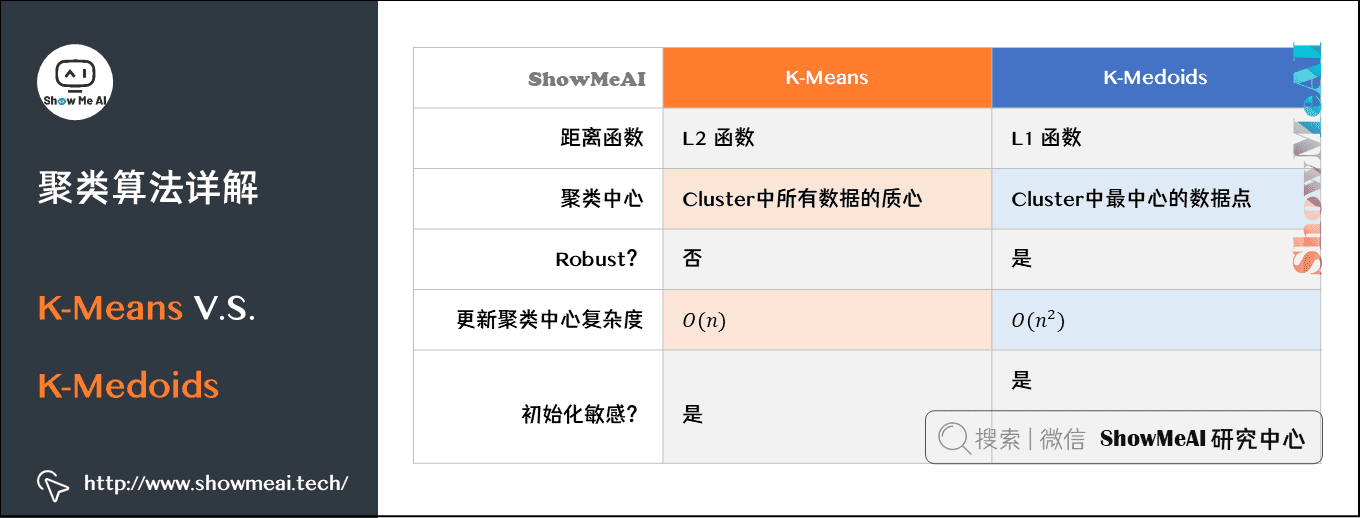# 4.层次聚类算法

相比于K-Means这类划分聚类，我们有另外一类层次化聚类算法。

## 1）层次聚类vs划分聚类

划分聚类得到的是划分清晰的几个类，而层次聚类最后得到的是一个树状层次化结构。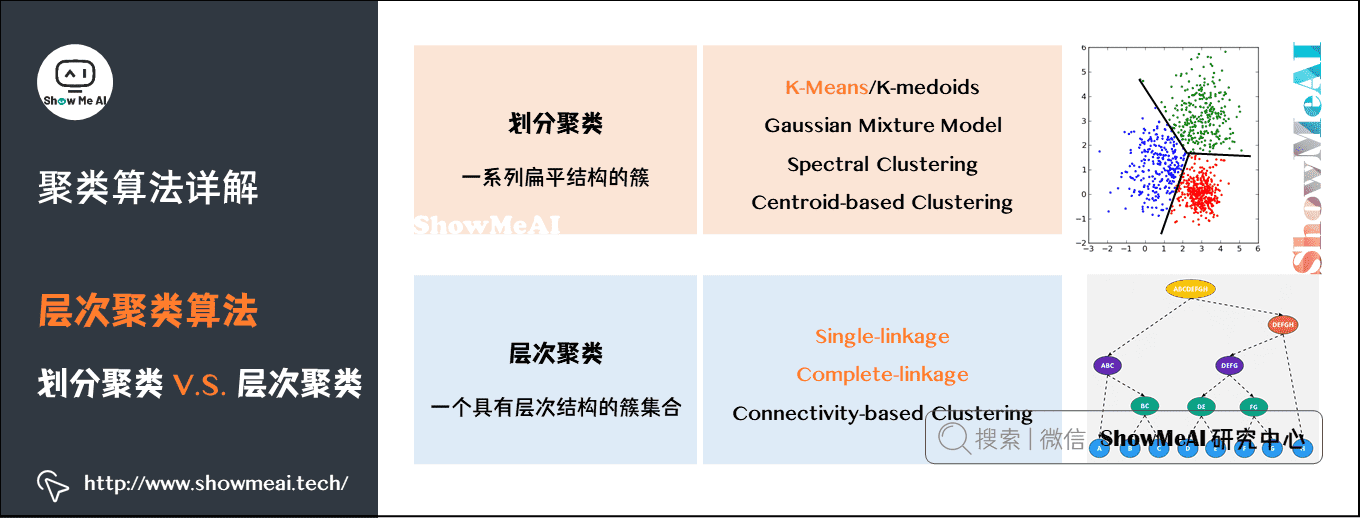从层次化聚类转换为划分聚类很简单，在层次化聚类的某一层进行切割，就得到1个划分聚类。如下图所示：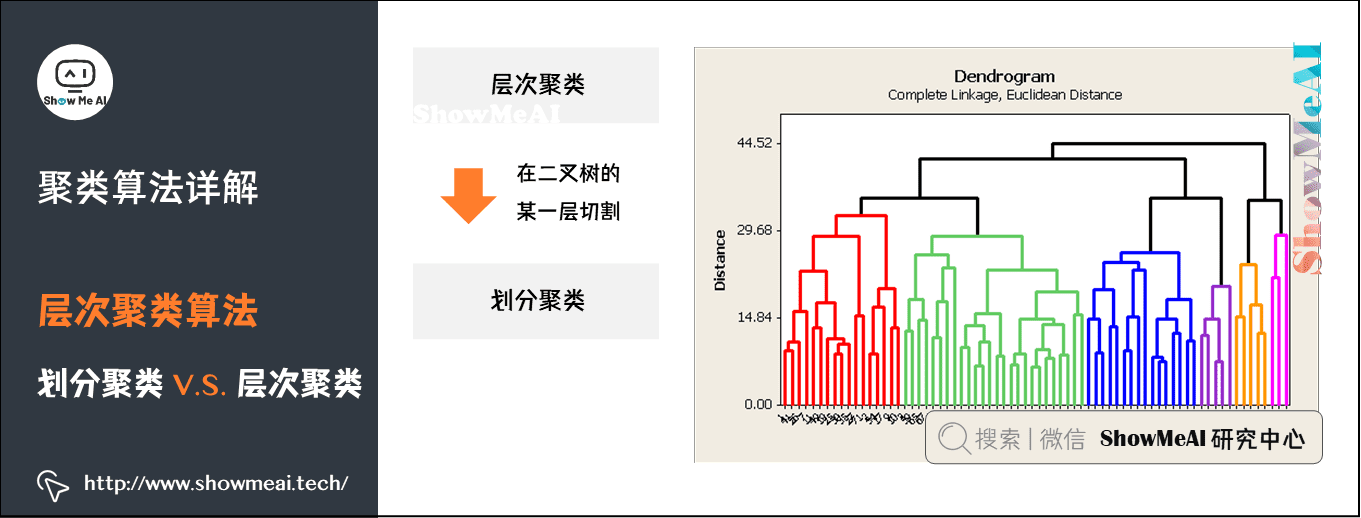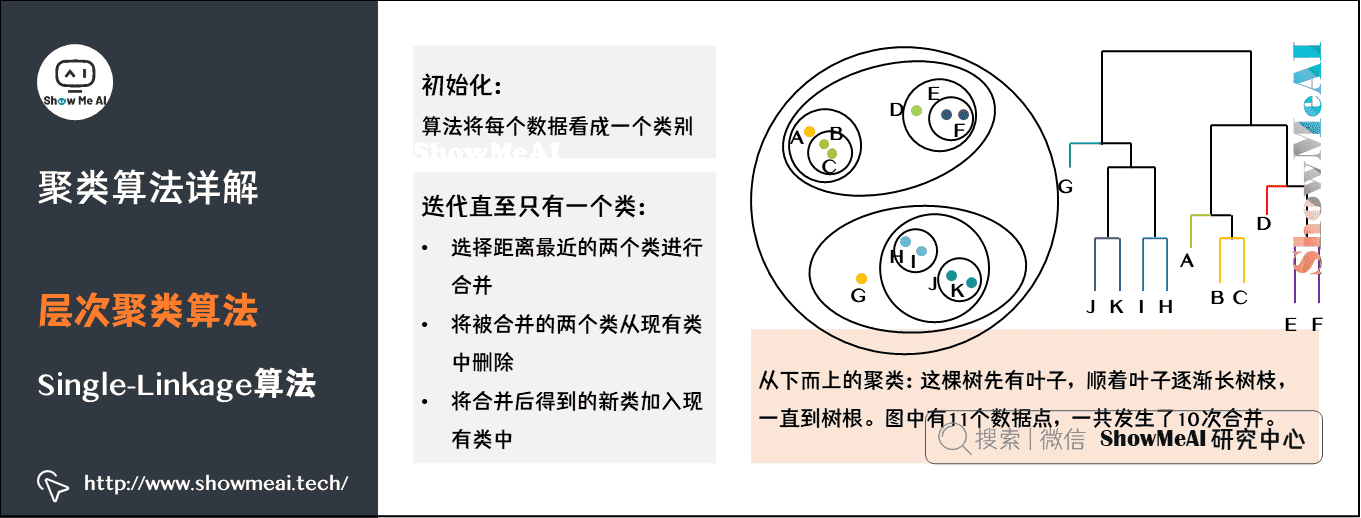这是一个从下而上的聚类。这棵树是先有叶子，顺着叶子逐渐长树枝，树枝越长越大一直到树根。

如果叶子很多，这个生长过程需要合并的类就会很多。图中有11个数据点，一共发生了10次合并。

这两种计算方法各有利弊。总的来说，层次聚类的计算复杂度是 O ( n 3 ) O(n^3) 级别，算是很高的了。可以用优先队列的数据结构对算法加速，加速后能减低到 O ( n 2 log ⁡ n ) O(n^{2} \log{n} ) 的级别。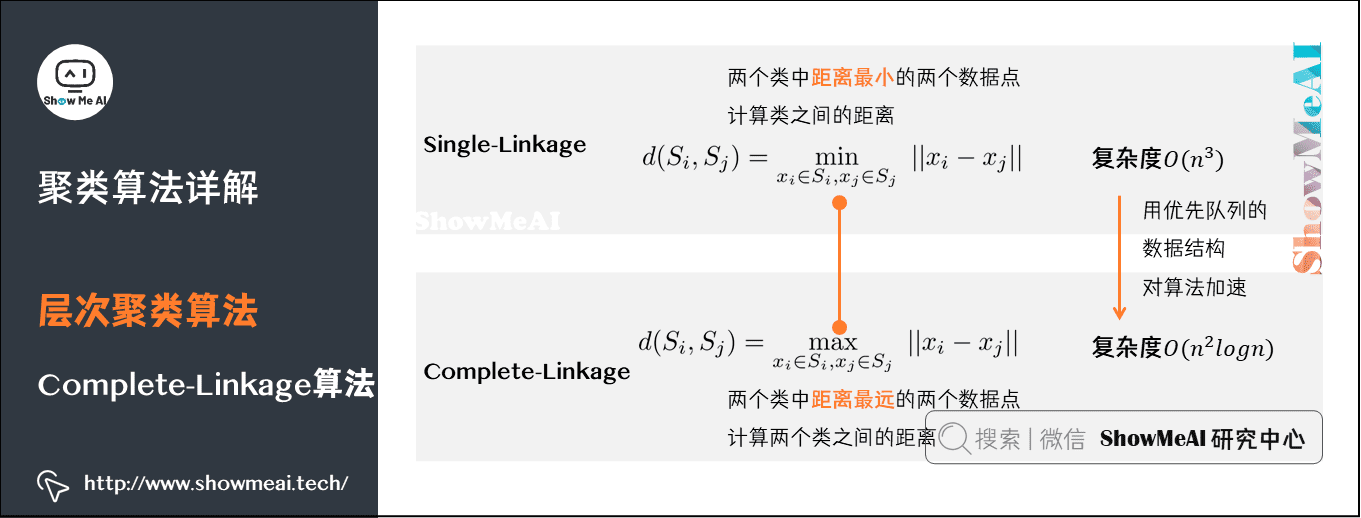# 5.DB-SCAN算法

## 1）DB-SCAN算法

在前面的内容中我们介绍了划分聚类和层次聚类的算法，接下来我们学习另外一个聚类算法：DB-SCAN算法。

DB-SCAN是一个基于密度的聚类。如下图中这样不规则形态的点，如果用K-Means，效果不会很好。而通过DB-SCAN就可以很好地把在同一密度区域的点聚在一类中。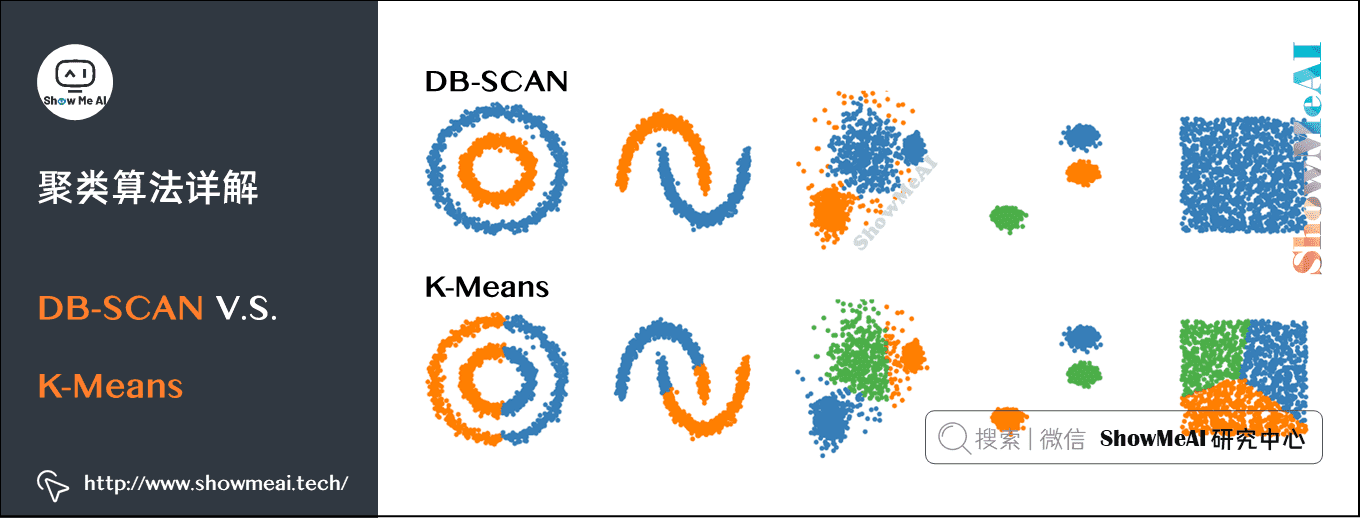## 2）DB-SCAN算法的关键概念

核心对象（Core Object），也就是密度达到一定程度的点。

• x j x_j ∈ − \in - 邻域至少包含MinPts个样本，即 ∣ N ∈ ( x j ) ∣ ≥ M i n P t s |N_\in (x_j )|≥MinPts ，则 x j x_j 是一个核心对象。

密度直达（directly density-reachable），密度可达（density-reachable）：核心对象之间可以是密度直达或者密度可达。

• x i x_i 位于 x j x_j ∈ − \in - 邻域中，且 x j x_j 是核心对象，则称 x j x_j x j x_j 密度直达。

• x i x_i x j x_j ，若存在样本序列 p 1 , p 2 , … , p n p_1,p_2, \dots, p_n ，其中 p 1 = x i p_1=x_i p n = x j p_n=x_j p i + 1 p_i+1 p i p_i 密度直达，则称 x j x_j x i x_i 密度可达。

密度相连（density-connected）：所有密度可达的核心点就构成密度相连。

• x i x_i x j x_j ，若存在 x k x_k 使得 x i x_i x j x_j ，均由 x k x_k 密度可达，则称 x i x_i x j x_j 密度相连。

我们通过下图深入理解一下刚才提到的几个基本概念。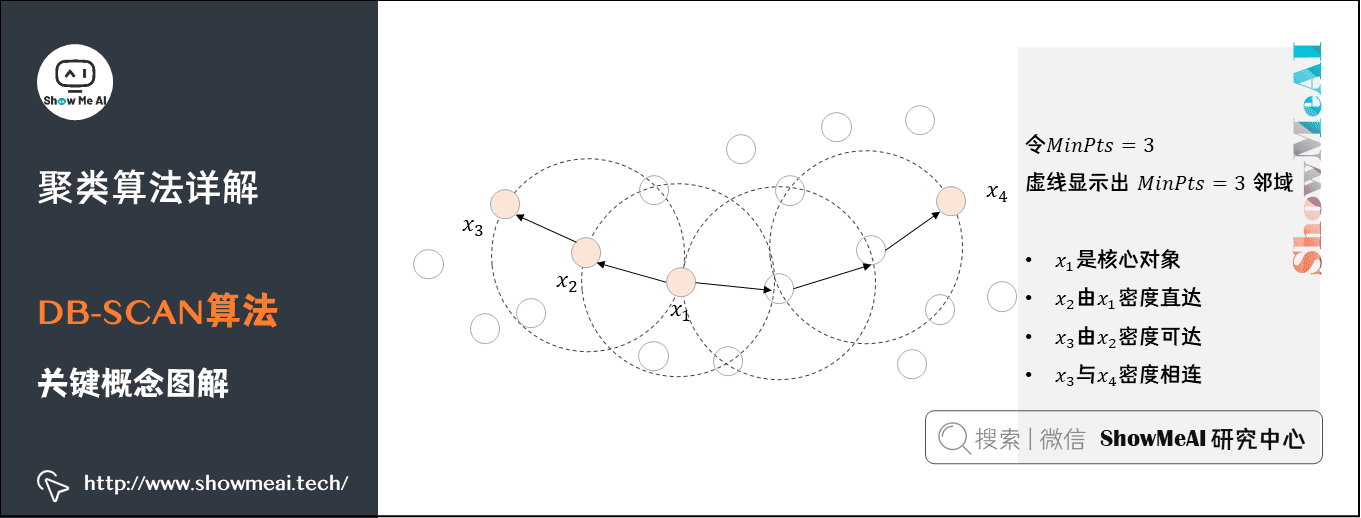先假设要求的最小点密度MinPts是3。

• 在一个半径范围内， x 1 x_1 这个点周围的点数是5，超过了阈值3，所以 x 1 x_1 是一个核心对象。同样， x 2 x_2 x 3 x_3 x 4 x_4 也是核心对象。

• x 1 x_1 x 2 x_2 处于一个邻域，所以二者是密度直达的关系，而 x 3 x_3 x 2 x_2 也是密度直达的关系，通过 x 2 x_2 x 1 x_1 x 3 x_3 是密度可达的关系。

• x 3 x_3 x 4 x_4 通过多个核心对象实现密度相连。

## 3）DB-SCAN算法伪代码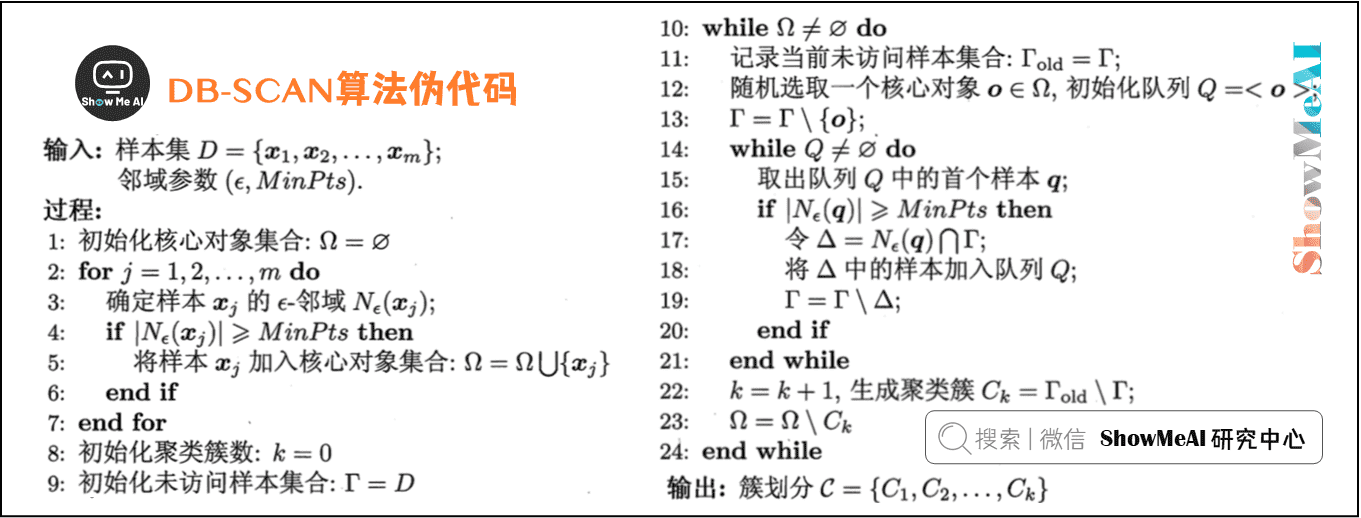这个过程用直白的语言描述就是：

• 对于一个数据集，先规定最少点密度MinPts和半径范围。

• 先找出核心对象：如果在半径范围内点密度大于MinPts，则这个点是核心对象。把所有的核心对象放到一个集合中。

• 从这个核心对象集合中，随机找一个核心对象，判断其它的数据点与它是否密度直达，如果是，则归入聚类簇中。

• 继续判断其它点与聚类簇中的点是否密度直达，直到把所有的点都检查完毕，这时候归入聚类簇中的所有点是一个密度聚类。

更多无监督学习的算法模型总结可以查看ShowMeAI的文章 AI知识技能速查 | 机器学习-无监督学习

## 视频教程

可以点击 B站 查看视频的【双语字幕】版本

【双语字幕+资料下载】MIT 6.036 | 机器学习导论(2020·完整版)

【双语字幕+资料下载】MIT 6.036 | 机器学习导论(2020·完整版)

https://www.bilibili.com/video/BV1y44y187wN?p=13

## ShowMeAI系列教程推荐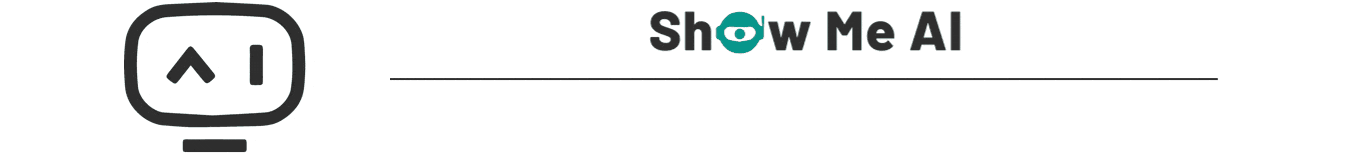展开全文聚类 算法 机器学习 kmeans 人工智能
• 在“无监督学习”中，训练样本的标记信息是未知的，目标是通过对无标记训练样本的学习来揭示数据的内在性质及规律，为进一步的数据分析提供基础。此类学习任务中研究最多、应用最广的是“聚类”(clustering) 聚类的...

# 聚类简介

在“无监督学习”中，训练样本的标记信息是未知的，目标是通过对无标记训练样本的学习来揭示数据的内在性质及规律，为进一步的数据分析提供基础。此类学习任务中研究最多、应用最广的是“聚类”(clustering)

聚类的目的是寻找一组一组相似的object，聚类希望类目内数据较近，类目之间的距离较远。

原型聚类

• K-means （对噪声敏感）
• 随机选取K个中心点
• 将数据分配到与之接近的中心点
• 使用数据均值去更新中心点，当中心点不再发生变化时停止
• 学习向量化
• 高斯混合聚类：使用概率模型来表示聚类效果

密度聚类（类似人眼分类）

• 核心对象：对象xj的ε−邻域中至少包含MinPts个样本Nε(xj)≥MinPts，则称xj为核心对象。
• 密度直达：若xj位于xi的ε−邻域中，且xi是核心对象，则称xj由xi密度直达。
• 密度可达：对xj与xi，存在样本序列p1,p2,...,pn且p1=xj,pn=xi,p1=xj,pn=xi 且pi+1由pi密度直达，则称xj由xi密度可达。
• 密度相连：对xj与xi，若存在xk使得xj与xi均由xk密度可达，则称xj由xi密度相连。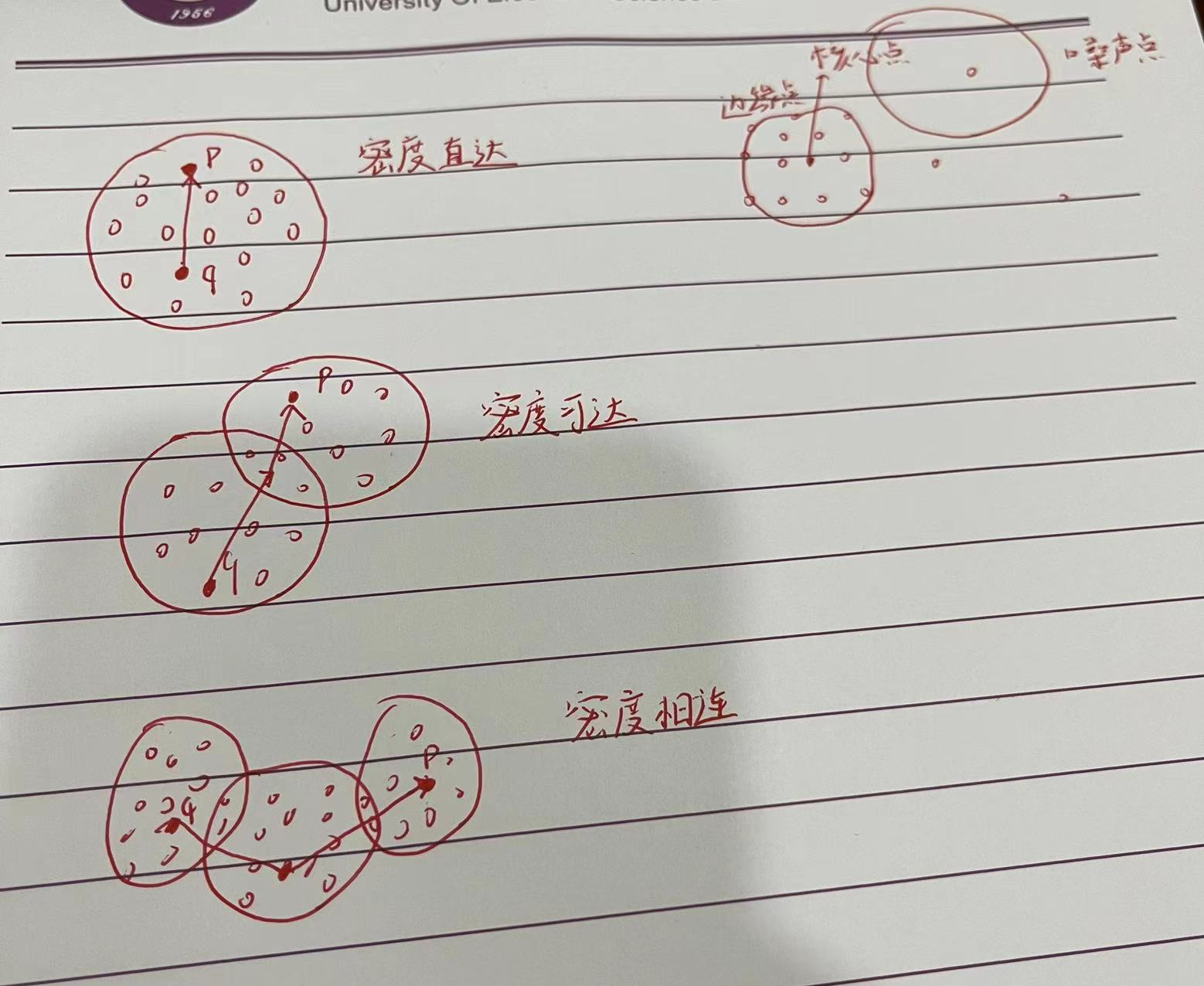DBSCAN将‘簇’定义为：由密度可达关系导出的最大的密度相连的集合。

层次聚类

层次聚类试图在不同层次对数据集进行划分，从而形成树形的聚类结构。数据集的划分可采用‘自底向上’的聚合策略，也可以采用‘自顶向下’的分拆策略。

AGNES是一种采用自底向上聚合策略的层次聚类算法。它将数据集中的每个样本看作一个初始聚类簇，然后算法运行的每一步找到距离最近的两个簇类进行合并，该过程不断重复，直到达到预设的聚类个数。这里的关键是如何计算聚类之间的距离，这里给出了三种距离。

最小距离：

最大距离：

平均距离：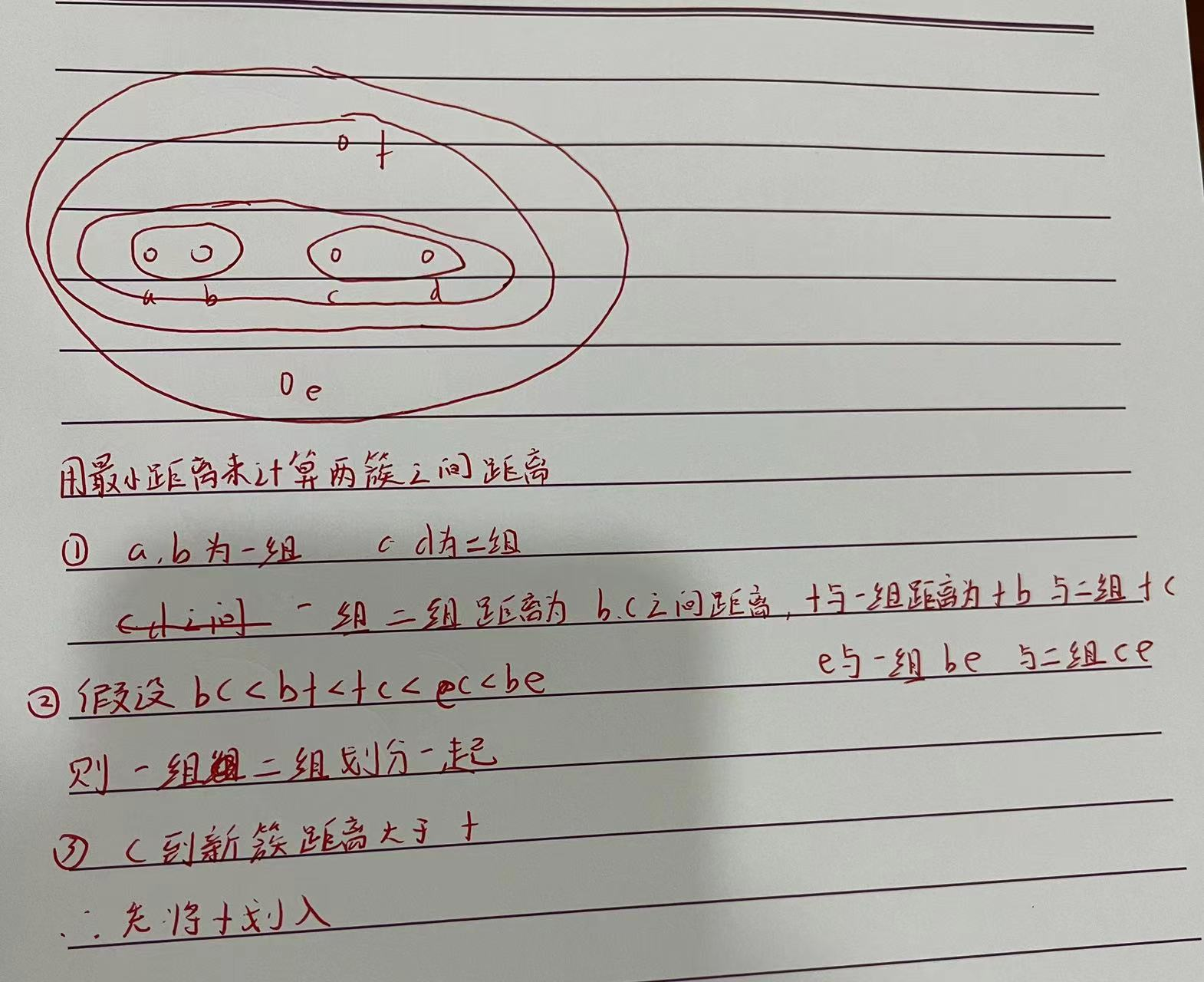# Sklearn实现聚类

机器学习代码KMeans（聚类）

展开全文python sklearn 聚类
• - 什么是聚类？ - 聚类与分类的区别 - Kmeans算法常用的距离公式 - Kmeans算法流程 - Kmeans算法实践 - Kmeans算法优缺点分析 - Kmeans算法应用场景

# 前言

我们之前遇到的所有机器学习算法都属于带标签的监督算法，而实现生活中大部分数据是不带标签的，从今天起我们要学一些处理不带标签数据的非监督算法，先从Kmeans聚类算法开始，我们将从以下几个方面来介绍Kmeans聚类算法

• 什么是聚类？
• 聚类与分类的区别
• Kmeans算法常用的距离公式
• Kmeans算法流程
• Kmeans算法实践
• Kmeans算法优缺点分析
• Kmeans算法应用场景

# 什么是聚类？

俗话说：“物以类聚，人以群分”，意思是说具有共同特质的人或物更容易聚集在一起，聚类算法正是依循这一客观规律，通过技术手段，将相似的研究对象聚集在同一个类里面，将相异的研究对象聚集在不同类里面，使得同一个类里面的研究对象具有极大的相似性，不同类的研究对象具有极大的差异性，聚类后的研究对象的内在结构就更加清楚明了。

# 聚类与分类的区别

聚类和分类看起来都是把一些样本分到相同或者不同的类，但是两者却有着本质区别，尤其是初学者对聚类和分类还是很难分清楚的，可以从以下几个方面来进行区分

(1) 目的

聚类的目的是要找出数据之间的相似性和相异性，在聚类之前并不清楚到底分多少类，也不清楚每个类里面包含哪些样本，甚至用什么方式方法来分也不确定，侧重总结归纳，而分类是在“分”之前就清楚知道总共有多少类，现在要确定新的样本到底属于哪个类，侧重预测。

(2) 操作

聚类是不需要利用已知数据进行训练的，直接聚类出结果，而分类是需要利用已有的数据训练出一个可靠的模型，然后利用这个模型去预测新的样本并给出判定，是一个训练加预测的过程；

(2) 范畴

正如上面所提，聚类是不需要利用已有的训练模型来对未知数据做预测，不需要数据类别标签，属于无监督学习，而分类是需要有一个训练过程得到一个训练模型，这个训练是有数据类别标签参与的，属于有监督学习。

# Kmeans算法常用的距离公式

数学上常用距离来度量两个对象之间相隔多远，除此之外，距离还可以用来表示研究对象之间的近似程度，而聚类算法就是要根据研究对象的相似程度来决定哪些应该聚在一起，哪些不应该聚在一起，在聚类算法里面常用的一些距离公式有欧几里得距离，曼哈顿距离，闵可夫斯基距离，切比雪夫距离，夹角余弦距离和杰卡德距离等。设 x ( i ) x^{(i)} x ( j ) x^{(j)} 是样本空间里的两个样本点，其分量分别如下

( x 1 ( i ) , x 2 ( i ) , . . . , x n ( i ) ) (x_1^{(i)}, x_2^{(i)},..., x_n^{(i)})

( x 1 ( j ) , x 2 ( j ) , . . . , x n ( j ) ) (x_1^{(j)}, x_2^{(j)},..., x_n^{(j)})

(1) 欧几里得距离

欧几里得距离，又叫欧式距离，是生活，研究中最常用的距离公式，用来表示欧式空间里面两个对象的远近程度， x ( i ) x^{(i)} x ( j ) x^{(j)} 的欧式距离可以用如下表达式来计算

d ( x ( i ) , x ( j ) ) = ∑ s = 1 n ( x s ( i ) − x s ( j ) ) 2 d(x^{(i)}, x^{(j)}) = \sqrt {\sum\limits_{s =1}^n (x_s^{(i)}- x_s^{(j)})^2}

Kmeans算法默认的就是欧式距离。

(2) 曼哈顿距离

曼哈顿距离也叫出租车距离或者城市距离，常用来刻画网格上面两点之间距离，其表达式如下

d ( x ( i ) , x ( j ) ) = ∑ s = 1 n ∣ x s ( i ) − x s ( j ) ∣ d(x^{(i)}, x^{(j)}) = \sum\limits_{s =1}^n |x_s^{(i)}- x_s^{(j)}|

(3) 闵可夫斯基距离

稍微复杂的距离公式还有闵可夫斯基距离公式，其表达式如下

d ( x ( i ) , x ( j ) ) = ( ∑ s = 1 n ( x s ( i ) − x s ( j ) ) q ) 1 / q d(x^{(i)}, x^{(j)}) =(\sum\limits_{s =1}^n (x_s^{(i)}- x_s^{(j)})^q)^{1/q}
可以看到曼哈顿距离是闵科夫斯基距离当q=1的特例，欧几里得距离是闵科夫斯基距离当q=2的特例。

(4) 切比雪夫距离

d ( x ( i ) , x ( j ) ) = max ⁡ 1 ≤ s ≤ n ∣ x s ( i ) − x s ( j ) ∣ d(x^{(i)}, x^{(j)}) = \max\limits_{1\leq s \leq n} |x_s^{(i)}- x_s^{(j)}|

由上式可以看到切比雪夫距离是闵科夫斯基距离的q趋向无穷大的极限形式，如果 x ( i ) x^{(i)} x ( j ) x^{(j)} 某些个分量差距很大，用切比雪夫距离有天然优势。

(5) 夹角余弦距离

如果把两个样本看成向量的话，可以计算向量之间的夹角，于是有了夹角余弦距离，一般的，如果夹角越小，相似度越高，反之，相似度越小，其表达式如下

c o s ( θ ) = ( x ( i ) , x ( j ) ) ∣ x ( i ) ∣ ∣ x ( j ) ∣ = ∑ s = 1 n x s ( i ) x s ( j ) ∑ s = 1 n ( x s ( i ) ) 2 ∑ s = 1 n ( x s ( j ) ) 2 cos(\theta) = \frac{(x^{(i)}, x^{(j)})}{|x^{(i)}||x^{(j)}|}=\frac{\sum\limits_{s =1}^n x_s^{(i)} x_s^{(j)}}{\sqrt{\sum\limits_{s =1}^n (x_s^{(i)})^2}\sqrt{\sum\limits_{s =1}^n (x_s^{(j)})^2} }

夹角余弦距离常用来刻画文本相似度。

(6) 杰卡德距离

如果是两个集合，不关心集合里面具体元素，还可以用杰卡德距离，先介绍杰卡德相似系数，假设现有集合A和集合B, 其杰卡德相似系数公式如下

J ( A , B ) = ∣ A ∩ B ∣ ∣ A ∪ B ∣ J(A,B) = \frac{|A\cap B|}{|A\cup B|}

杰卡德相似系数表示两个集合的交的元素占两个集合的并的元素的比例，特别的，A与B完全相同，则 ∣ A ∩ B ∣ = ∣ A ∪ B ∣ |A\cap B| = |A\cup B| ，则J(A,B)=1

而杰卡德距离是在杰卡德系数的基础上再进一步加工

d i s t ( A , B ) = 1 − J ( A , B ) = ∣ A ∪ B ∣ − ∣ A ∩ B ∣ ∣ A ∪ B ∣ dist(A,B) = 1 - J(A,B) = \frac{|A\cup B|-|A\cap B|}{|A\cup B|}

dist(A,B)表示A与B之间的距离，距离越小，相似度越高，反之，相似度越低。

# Kmeans算法流程

Kmeans算法包括簇分配和质心移动两个基本步骤，具体的

(1)初始化K个质心；

(2)计算每个样本到K个质心的距离，把每个样本分配予最近的质心，完成簇分配，记录此时每个样本的簇标签和整体损失函数；

(3)重新计算每一簇的质心，移动质心，同时，计算此时的整体损失函数；

(4)如果(3)的整体损失函数比(2)的整体损失函数小，重复第二步第三步，直到整体损失函数不再下降为止；

Kmeans算法流程如下

# Kmeans实践

我们以吴恩达机器学习第七次作业为例子来进行实践，详细具体的掌握Kmeans算法。

## 读取数据并可视化

原始数据能够可视化的尽量可视化，原始数据可视化最大的好处就是非常直观的看到数据分布，猜到最佳聚类的数目和最后的大致效果。

import math
import numpy as np
import pandas as pd
import scipy.io as scio
import matplotlib.pyplot as plt

#数据可视化
X = data["X"]  #50*2维数组
plt.figure(figsize=(6,4)) #新建画布
plt.scatter(X[:,0], X[:,1]) #散点图
plt.xlabel("X1")
plt.ylabel("X2")
plt.show()## 簇分配和质心移动

利用散点图知道聚多少类之后，就有的非常关键参数K，接下来要做的就是严格按照Kmeans算法流程中的簇分配和质心移动。

### 初始化K个质心

实践表明第一步K个质心的选择好坏直接关系到最终的收敛效果和收敛步数，在初始质心的选择上面有两个关键因素需要考虑

一个是随机性

随机性是指初始质心的选择可以有很多种可能，可以是原样本点，也可以是非原样本点，一般选择原样本点比较好。

一个是离散性

虽然随机性可以让初始质心有很多种选择，但是质心又有牵引的作用，为保障最终收敛效果和收敛步数，初始质心还应该尽可能离散。

initial_centroids = X[np.random.permutation(len(X))[0:K],:] #随机取K个样本作为初始质心


这行代码是将原样本空间随机打乱再取打乱后样本空间的前面K个，相当于从原样本空间随机抽取了K个样本。

### 把每个样本分配予最近的质心

有了初始K个质心，就可以计算每一个样本到这些质心的距离，这样每个样本就会产生K个距离，可以比较这K个距离的大小，选出最小距离对应的那个质心，并将该样本划归到这个质心簇里，并标记该样本的所属的簇号，这样每个样本也有了一个簇标签，完成了第一轮簇分配。

def find_closet_centroids(myX, my_init_centroids): #给出初始聚类中心点，计算每个样本到中心点的距离并划分类
m = myX.shape #样本数
K = my_init_centroids.shape #聚类个数
idx = np.zeros([m, 1]) #用来存放簇中心
for i in range(m): #对每一个样本循环
distance = [] #用来存放距离，每一个样本有k个距离
for j in range(K):
#print(myX[i,:], centeriods[j,:], myX[i,:] - centeriods[j, :])
dist = np.linalg.norm(myX[i,:] - my_init_centroids[j,:]) #每个样本都有k个距离，二阶范数即欧氏距离
distance.append(dist)
idx[i] = np.argmin(distance) #k个距离中取最小的
return idx


### 重新计算每一簇的质心，移动质心

每个样本都有了自己所属的簇标签后，就可以重新计算该簇的质心，一般以该簇的算术平均值作为新的质心，该簇的质心移动到新的质心上。

def compute_centroids(myX, myidx): #重新计算簇中心
m = myX.shape #样本数
n = myX.shape[-1] #特征数
K = 3 #簇数
centroids = np.zeros((K, n)) #簇*特征数,如同前面的initial_centroids
counts = np.zeros((K, n)) #簇*特征数,用来存放各簇的样本数
for i in range(m):
centroids[int(myidx[i])] += myX[i] #簇求和
counts[int(myidx[i])] +=1 #簇计数
new_centroids = centroids/counts #簇平均值
return new_centroids


### 判定移动质心是否对结果提升

在评价质心的移动对聚类结果是否有提升需要一个评价尺度，也就是要设计一个整体损失函数来刻画每一步的操作是否对结果是否有提升，如果有提升，那么就沿着这个方向继续进行下去，如果没有提升就要考虑停下来，或者转变方向。在设计整体损失函数时候，需要考虑样本情况，也需要质心情况，以及前后可对比性。Kmeans聚类算法的整体损失函数可以设计成

C o s t ( c 1 , . . . , c m , u c 1 , . . . , u c K ) = 1 m ∑ i = 1 m ( x ( i ) − u c i ) 2 Cost(c_1,...,c_m, u^{c_1},...,u^{c_K}) = \frac{1}{m}\sum\limits _{i=1}^m (x^{(i)} -u^{c_i})^2

其中， c i c_i 代表样本 x ( i ) x^{(i)} 被分配的簇标签， c i ∈ { 1 , 2 , . . . , K } c_i\in \{1,2,...,K\} μ c i μ^{c_i} 代表 x ( i ) x^{(i)} 被分配簇标签所对应的质心。 整体损失函数是一个收敛函数，如果下一轮算出的值比上一轮的值小，说明质心的移动确对结果有所提升，反之，质心移动没有对结果提升，达到收敛状态。

def cost(myX, myidx, centroids): #计算损失函数
cost_value = 0
for i in range(len(myX)):
cost_value += np.sum((X[i] - centroids[int(myidx[i])])**2)
cost_value /= len(myX) #整体损失值
return cost_value


### 直至收敛

有了前面几小步的准备工作，现在要做的就是将其封装起来，给出整体损失函数，并设置收敛条件让其一步一步的去进行簇分配和质心移动工作，直至收敛为止。

def run_kmeans(myX, K): #在样本上运行kmeans算法
m = myX.shape #样本数
n = myX.shape #特征数
centroids = [] #用来存放每一步的簇中心
cost_values = [] #用来存放每一步的整体损失值
initial_centroids = myX[np.random.permutation(m)[0:K],:] #随机取K个样本作为初始中心点
centroids.append(initial_centroids) #把初始中心点追加进去
initial_idx = find_closet_centroids(myX, initial_centroids) #计算每个样本到中心点的距离，并返回类别标签
initial_cost_value = cost(myX, initial_idx, initial_centroids) #计算第一次的整体损失函数
cost_values.append(initial_cost_value) #把第一次整体损失函数追加进去
new_centroids = compute_centroids(myX, initial_idx) #重新计算聚类中心
centroids.append(new_centroids) #把新的聚类中心追加进去
new_cost_value = cost(myX, initial_idx, new_centroids) #重新计算整体损失函数,此时只是质心改变，类别标签并没改变
cost_values.append(new_cost_value) #把新损失函数值追加进去
while cost_values[-2]>cost_values[-1]: #判定损失函数值是否降低
idx = find_closet_centroids(myX, centroids[-1]) #以新的质心进行簇分配
centroid = compute_centroids(myX, idx)
cost_value = cost(myX, idx, centroid)
centroids.append(centroid)
cost_values.append(cost_value)
return idx, centroids, cost_values


## 聚类过程可视化

虽然前面实现了聚类模型，如果能够将聚类过程可视化呈现一定是锦上添花，更加能够明白聚类原理。

### 质心分割

由于之前的质心是以数组统一保存起来，但是在绘制质心移动轨迹的时候并不好用，所以需要做一个质心分割工作，简单的来说就是将所有质心分成K组，代表K个簇，每一组都有迭代次数个质心点坐标， 这样没一簇的质心移动就清晰了

def centroid_split(mycentroids, K): #将所有中心点分簇
step = len(mycentroids) #收敛步数
cluster_center = []#用来存放各簇的中心点坐标
for k in range(K):
for i in range(step):
cluster_center.append(tuple(mycentroids[i][k]))
cluster_center_k = [cluster_center[i:i+step] for i in range(0, len(cluster_center), step) ]  #每簇的簇中心点坐标
return cluster_center_k


### 质心移动轨迹和簇分布

这一部分需要将最后收敛状态下每簇的样本分布区分开来，并且把各簇的质心移动轨迹绘制出来

def visual_Kmeans(myX, K): #可视化Kmeans过程
plt.figure(figsize=(6,4)) #新建画布
plt.subplot(1,2,1) #第一个子图
plt.xlabel("X1")
plt.ylabel("X2")
idx, centroids, cost_values = run_kmeans(myX, K)
plt.scatter(myX[:,0], myX[:,1], c = idx) #根据idx标签绘制各簇散点图
cluster_center = centroid_split(centroids, K) #中心点分簇
for k in range(K): #绘制K个簇中心移动轨迹
centroid_x = [] #用来存放每簇的中心点的每一步的x1值
centroid_y = [] #用来存放每簇的中心点的每一步的x2值
cluster_xy = cluster_center[k]
for s in range(len(cluster_xy)):
centroid_x.append(cluster_xy[s])
centroid_y.append(cluster_xy[s])
plt.plot(centroid_x, centroid_y, '-->' , linewidth = 0.7)
plt.subplots_adjust(left=None, bottom=None, right=None, top=None, wspace=0.3, hspace=0.2) #调整子图间距
plt.subplot(1,2,2) #第二个子图
plt.plot([i for i in range(len(cost_values))], cost_values) #损失函数折线图
plt.xlabel("iteration")
plt.ylabel("cost")
plt.show()


# Kmeans算法优缺点分析

• 优点

(1) 适用性强

很多未知特性的数据集都可以先用K-means去试试，探索数据内在结构。

(2) 过程简单

• 缺点

Kmeans聚类算法的缺点也很明显，如

(1) 需要事先知道聚类数目；

参数K作为一个输入参数，是在聚类之前就要根据业务或者经验来确定；

(2) 初始质心选择影响很大；

因为初始质心选择上要具备随机性，存在一些不确定因素，并不能保证每一次初始化聚类操作都能开始于一个合适的状态，不恰当的初始质心可能使得损失函数在聚类过程中陷入局部最小值，达不到全局最优的状态，下图是多次初始质心选择聚类结果和损失函数收敛情况。从上图可以看到前3次聚类并没有达到全局最优化，这正是因为初始质心的选择不当导致的。

# Kmeans算法应用场景

Kmeans聚类算法的应用场景很多，凡是一些不代标签的数据需要聚类的都可以用Kmeans算法试一试。这里重点介绍一下Kmeans在反欺诈领域的应用，在反欺诈领域经常利用过往欺诈性索赔的黑数据，根据数据的相似性来提炼欺诈方式和欺诈模式，还可以识别新的欺诈行为。

# 参考文献

1, permutation用法

https://blog.csdn.net/ljyljyok/article/details/102929918

2, 调整子图间距

https://blog.csdn.net/zengbowengood/article/details/103279583

3, 多张静态图变成动图imageio

https://blog.csdn.net/zengbowengood/article/details/109336369展开全文• **K-均值**是最普及的聚类算法，算法接受一个未标记的数据集，然后将数据聚类成不同的组。 **K-均值**是一个迭代算法，假设想要将数据聚类成n个组，其方法为: 1. 首先选择$K$个随机的点，称为**聚类中心**（**...深度学习 机器学习 算法
• 在本教程中，您将使用无监督学习来发现数据中的分组和异常点。在无监督学习中，没有用于显示期望结果的真值(ground truth) 或带标签的数据集。而是获取原始数据并使用各种算法来发现数据集群。如果您想了解无监督...
• 在“无监督学习”中，训练样本的标记信息是未知的。目标是通过对无标记训练样本的学习来揭示数据的内在性质及规律，为了进一步的数据分析提供基础机器学习 聚类
• 而本次学习聚类，对于很多样本而言，他们没有分类标签，我们可以通过某个特定标准，使同一个簇内的数据对象的相似性尽可能大，同时不在同一个簇中的数据对象的差异性尽可能地大，我们不关心数据具体属于哪一类，...聚类 机器学习 算法
• 机器学习 聚类分析 复旦大学 赵卫东 博士 wdzhao@ 章节介绍 聚类分析是一种典型的无监督学习用于对未知类别的样本进行划分将 它们按照一定的规则划分成若干个类族把相似(距高相近)的样本聚在同一 个类簇中把不相似的...
• 聚类是一种非监督式学习算法，它不要求源数据集有标签，一般应用于做数据探索性分析，聚类算法的结果是将不同的数据集按照各自的典型特征分成不同类别，不同人对聚类的结果解读可能不同。 总体上来说，聚类算法分为...机器学习 聚类 K-Means 层次聚类 划分聚类
• 讲解什么是聚类，性能度量与距离度量，原型聚类，层次聚类，密度聚类机器学习 机器学习算法 机器学习实战 python机器学习
• 本文为大家分享了Python机器学习之K-Means聚类的实现代码，供大家参考，具体内容如下 1.K-Means聚类原理 K-means算法是很典型的基于距离的聚类算法，采用距离作为相似性的评价指标，即认为两个对象的距离越近，其...
• 目录理论部分1.1 提出背景1.2...该聚类算法同样也是基于密度聚类的算法，与DBSCAN不同的是，该算法的设计使得其对初始超参数的设定敏感度较低。 1.2 OPTICS算法 1.2.1 基本概念 ·核心距离 一个对象ppp的核心距离定义为算法 机器学习 聚类
• 首先复习了无监督学习的内容以及聚类算法的应用。其次从直观上介绍了 K 均值算法，以及该算法的规范表达和具体的应用（分离不佳的簇）。在优化目标的部分提到了失真代价函数，同时也对 K 均值算法进行了补充。随机...聚类 机器学习 算法
• 聚类概念 聚类是把相似的东西分到一组，它是一个无监督问题，没有标签使用 难点： 对于有标签的有监督学习问题，标签可以便于我们来评估模型，无监督学习问题在评估上比较难一点 对于不同的参数组合，得到的学习结果...
•人工智能 机器学习 聚类
• 实质上是机器学习中的一种无监督学习方法。并不需要我们对数据进行标记。通常，它被用作在一组示例中找到有意义的结构、解释性基础过程、生成特征、进行分组的等。 聚类是将总体或数据点划分为多个组的任务，以使同...机器学习 无监督学习 聚类 高斯混合模型
• 聚类机器学习中一种重要的无监督算法，它可以将数据点归结为一系列特定的组合。理论上归为一类的数据点具有相同的特性，而不同类别的数据点则具有各不相同的属性。在数据科学中聚类会从数据中发掘出很多分析和理解...聚类 算法 机器学习
• 文章目录参考资料1. 基本概念1.1 距离度量1.2 性能度量1.2.1 外部指标1.2.2 内部指标 参考资料 Machine-learning-learning-notes ...聚类是一种经典的无监督学习方法，无监督学习的目标是通过对无标记训机器学习 人工智能 聚类
• 无监督学习是机器学习的一种方法，没有给定事先标记过的训练示例，自动对输入数据进行分类或分群。 无监督学习的优点： ①算法不受监督信息（偏见）的约束，可能考虑到新的信息。 ②不需要标签数据，极大程度上扩大...机器学习
• 一、 机器学习概述 1.1 监督学习与无监督学习 监督学习：基于给定的数据数据与分类训练分类器以期达到比较好的分类效果。（Logistic回归、决策树、SVM） 无监督学习：根据数据进行建模，对样本进行分类（通过对...
• 与监督学习中的性能度量作用类似，对聚类结果，我们需要通过某种性能度量来评估其好坏；另一方面，若明确了最终将要使用的性能度量，则可直接将其作为聚类过程的优化目标，从而更好地得到符合要求的聚类结果。 聚类...聚类 机器学习 python
• 机器学习实践：足球比赛聚类分析 1、实验描述 本实验利用K-Means聚类分析算法对足球比赛结果进行分析，该算法通过Sprak Mllib库来调用，我们将学习K-Means算法的K值选取，聚类原理等内容，理解聚类算法在实际业务...机器学习 聚类 人工智能
• 机器学习入门聚类算法 1、实验描述 本实验先简单介绍了一下各聚类算法，然后利用鸢尾花数据集分别针对KMeans聚类、谱聚类、DBSCAN聚类建模，并训练模型；利用模型做预测，并使用相应的指标对模型进行整体的评估...机器学习 聚类 算法
• 聚类 K-MEANS KMEANS代价函数 能够帮助我们调试学习算法，确保k均值聚类算法是在正确运行中； 能够运用代价函数帮助k-均值找到更好的簇，并且避免局部最优解 聚类的过程为减小代价函数的过程，代价函数为： J = min...聚类 算法 python
• 在现实生活中，很少直接用聚类算法，因为聚类效果的好坏不容易衡量（因为没有标记，就没有标准答案），有时候会用做监督学习中稀疏特征的预处理，把混乱的数据先分分看，看看大类如何。 算法思想   聚类算法，...机器学习 Python 聚类算法
• 本博客为唐宇迪老师python数据分析与机器学习实战课程学习笔记 一.聚类 给定一组数据点，我们可以使用聚类算法将每个数据点划分为一个特定的组。理论上，同一组中的数据点应该具有相似的属性和/或特征，而不同组中...K-MEANS 机器学习 DBSCAN...